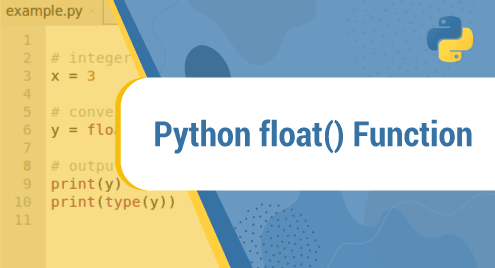Scripting

# Python float() Function## Introduction

The float() function in Python converts a number or a string and returns a floating-point number. The function works with a number that can be an integer or a decimal. A string can contain any number, can contain mathematical operators, and can be used with NaN, Infinity, or inf.

Now we’re gonna guide you about using the float() function in Python. Hope you understand.

## Example

```x = float(6)

print(x)```

Output:

6.0

## Definition

The float() function converts a specific number to a floating-point number.

## The syntax

`float(value)`

Parameter Values:

value: can be a number or a string

## More examples

Example 1: Converting an integer to a float

```integer = 60

x = float(integer)

print(x)```

Output:

60.0

Example 2: Converting a string to a float

```string = 0.500

x = float(string)

print(x)```

Output:

0.5

Example 3: for infinity and Nan

```x = "Inf"

y = "nan"

print(float(x))

print(float(y))```

Output:

inf

nan

## Conclusion

And we guided you on how to use the float() function in Python.

Thank you for checking it out!